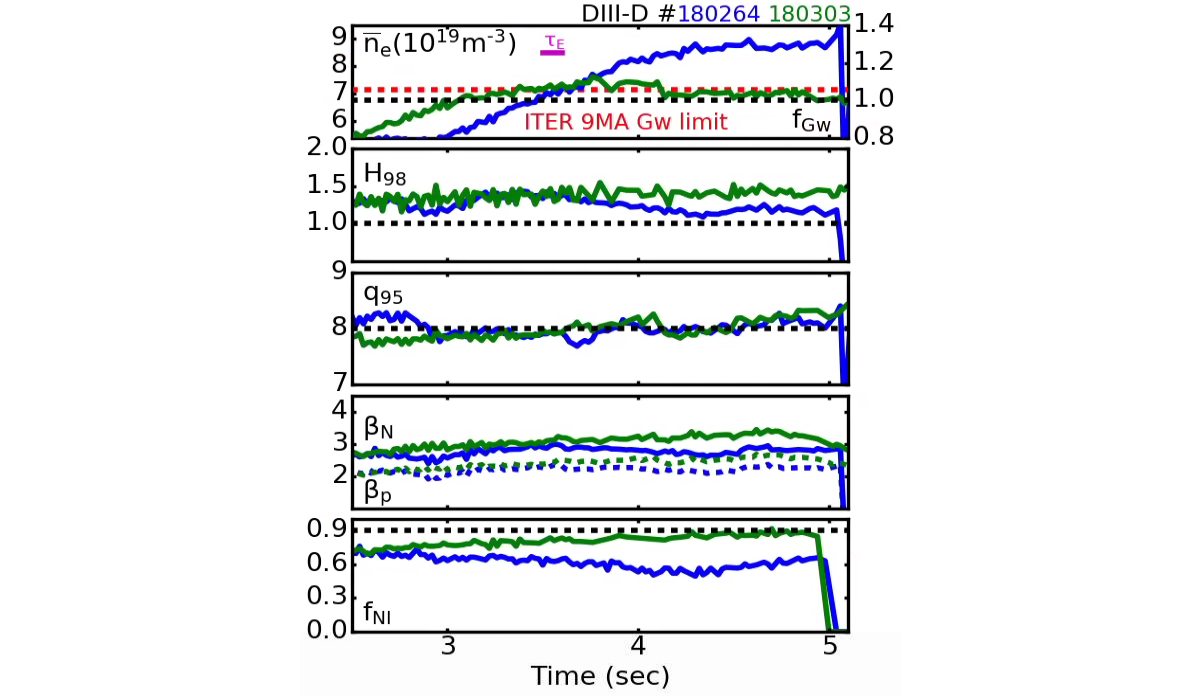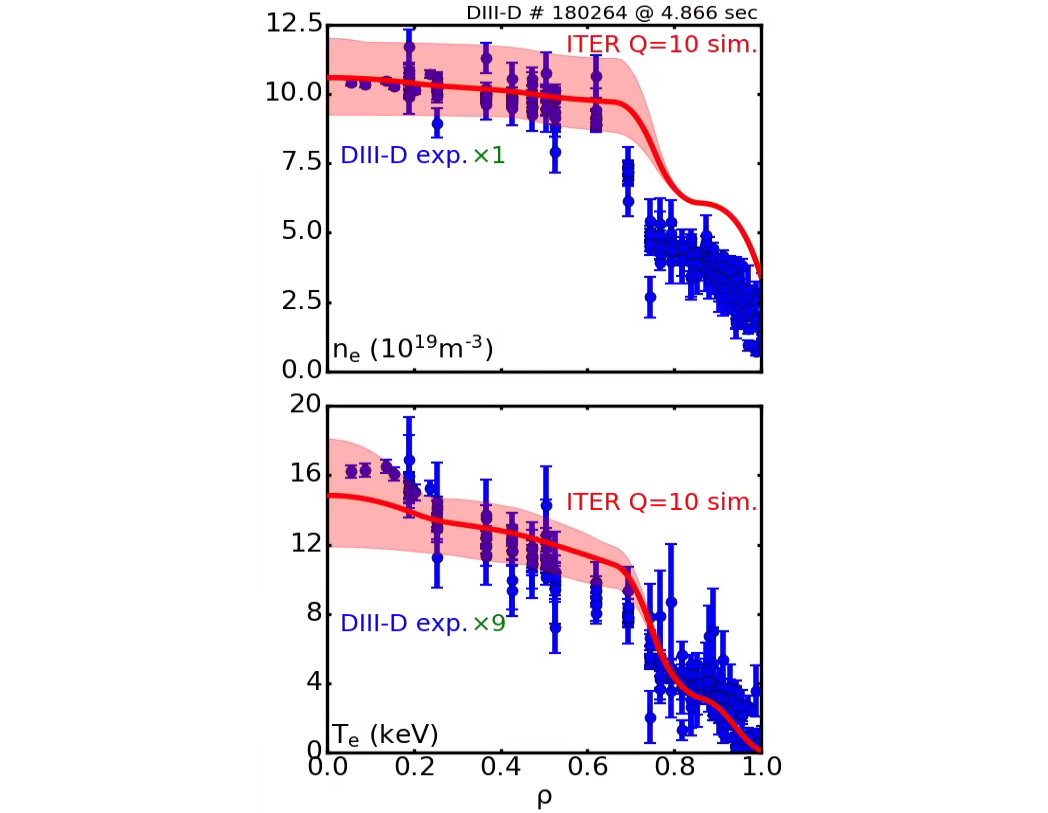Since 18 of December 2019 conferences.iaea.org uses Nucleus credentials. Visit our help pages for information on how to Register and Sign-in using Nucleus.

# 28th IAEA Fusion Energy Conference (FEC 2020)

May 10 – 15, 2021
Virtual Event
Europe/Vienna timezone
The Conference will be held virtually from 10-15 May 2021

## A Low Plasma Current (~ 8 MA) Approach for ITER’s Q=10 Goal

May 11, 2021, 9:04 AM
17m
Virtual Event

#### Virtual Event

Regular Oral Magnetic Fusion Experiments

### Speaker

Siye Ding (ORAU/ASIPP)

### Description

Experiments on DIII-D support a new approach, confirmed by transport modeling, to achieving Q=10 in ITER using a scenario with low plasma current (~ 8 MA), high $\beta_{\rm p}$, and line-averaged Greenwald fraction ($f_{\rm Gw}$) above 1. At 8 MA the disruption risk and ELM challenge are greatly reduced, with the possibility that uncontrolled ELMs may be acceptable$^1$. Due to the need of sufficient fusion power and low plasma current, this approach requires high density with $f_{\rm Gw}$>1.0 simultaneous with high confinement quality ($H_{\rm 98y2}$>1). Using impurity injection, the recent DIII-D experiments achieve and maintain these simultaneous conditions. Previously, high $\beta_{\rm p}$ plasmas with $f_{\rm Gw}$ up to ~1.0 and $H_{\rm 98y2}$>1 were obtained in JT-60U, albeit transiently and usually operated at low absolute density$^{2,3}$, which is not favorable to reactor plasma. For the first time in a tokamak, experiments demonstrate that a stationary ITB at large radius ($\rho$~0.7) is compatible with $H_{\rm 98y2}$>1, at reactor-level absolute density ($n_{\rm e0}>1.0×10^{20}\;m^{-3}$), $f_{\rm Gw}$>1, and reactor-relevant $q_{95}$ as well (fig. 1). Such ITB is a key feature of the ITER 8 MA Q=10 modeling. Comparison between the experimental DIII-D profiles and the predicted ITER profiles shows also a good match of ITB location and profile shape (fig. 2). The DIII-D experiments confirm that the high density ITB in ITER modeling is achievable at similar $q_{95}$ using the high $\beta_{\rm p}$ scenario.For the first time, neon injection is observed to trigger the formation of a large radius ITB in the density channel at reactor level density on DIII-D, which provides an effective experimental approach to achieve line-averaged Greenwald fraction well above 1. Pedestal density feedback control is used in the experiment. Therefore, the pedestal density is kept below the Greenwald limit, e.g. $f_{\rm Gw,ped}$<0.7. The large radius ITB strongly elevates the plasma density inside the pedestal. The density profile is fairly flat inside the ITB with $n_{\rm e0}>1.0×10^{20}\;m^{-3}$ (fig. 2). These high $\beta_{\rm p}$ experiments on DIII-D also show that the ITB is sustained long after (>8×the energy confinement time, $\tau_{\rm E}$) the neon injection is turned off. Impurity transport modeling based on the experimental data shows that neon provides electron source at $\rho$~0.75, which is the location where the foot of the ITB emerges. One of the possible mechanisms for the trigger of ITB formation could be that the important electron source creates a seed of local density gradient. The locally increased density gradient strengthens the effect of $\alpha$-stabilization of turbulence by reducing the non-adiabaticity of electron response$^4$, and starts a positive feedback leading to ITB formation. The same technique may also work for ITER high $\beta_{\rm p}$ plasma, when higher Z impurity is employed.Using this technique, new high $\beta_p$ experiments on DIII-D demonstrate the stationary large radius ITB at reactor level density and reactor relevant $q_{95}$. Fig. 1 shows that the stationary phases of $f_{\rm Gw}$~1.0-1.1 and $f_{\rm Gw}$~1.3 at $q_{95}$~8 are sustained for 21 and 8 $\tau_{\rm E}$’s, respectively. These plasmas have even higher density than ITER at its 9 MA Greenwald limit. With ITB, the energy confinement is well above standard H-mode in these plasmas, e.g. $H_{\rm 98y2}$ up to 1.4. The degradation of confinement in one of the discharges shown in fig. 1 ($f_{\rm Gw}$~1.3 case) is due to excessive neon injection causing very high core radiation. The averaged neon injection rates are $1.3×10^{20}$ /s ($f_{\rm Gw}$~1.3) and $4.8×10^{19}$ /s ($f_{\rm Gw}$~1.1). With further optimization of the impurity injection waveform, sustained high confinement is also expected in the $f_{\rm Gw}$~1.3 case. These high $\beta$ ($\beta_{\rm N}$≤3.5, $\beta_{\rm p}$≤2.7) plasmas have $q_{\rm min}$>2.0 and quiet MHD behavior. Meanwhile, a non-inductive current fraction up to 0.9 is achieved simultaneously in the high density phase.

1D Transport modeling suggests the goal of Q=10 on ITER can be achieved at low plasma current (~ 8 MA) using the high $\beta_{\rm p}$ scenario. The simulations are performed using the STEP module in the OMFIT framework, integrating sub-modules for transport, heating, current drive and equilibrium calculations. Compared to previous ITER simulation works, the innovative features in this work include: 1) Plasma density, temperature, current profiles and equilibrium are all evolved in the simulations, while pedestal heights for density and pressure are prescribed to values slightly below the Greenwald limit (for density) and EPED prediction (for pressure); 2) Use TGLF model for transport prediction; 3) In TGYRO, E×B shear effect on turbulence suppression is turned off. Simulations use ITER “Day One” heating and current drive power: NBI≤33 MW, EC≤20 MW. Calculations predict that the following parameters can be achieved: Q=9.5±2.5, $I{\rm p}$=7.85±0.35 MA, $q_{95}$=7.54±0.39, $H_{\rm 98y2}$=1.74±0.13, $f_{\rm Gw}$=1.48±0.13, $f_{\rm NI}$≥98% and $P_{\rm fus}$=350±50 MW. A large radius ITB is shown in both temperature and density channels for electron and ion species (fig. 2).

Density profiles experimentally achieved on DIII-D match the density profiles simulated for ITER Q=10 in shape (ITB radius) and absolute core density value (fig. 2), while the core confinement quality is maintained well above standard H-mode levels. Although the experimental electron temperature is low compared with ITER simulation results, its shape is still a very good match to the ITER simulation results, if a multiplier of 9 is applied to the DIII-D data. The results confirm that the required large radius ITB at $f_{\rm Gw}$ above 1 and reactor level density in ITER Q=10 modeling is achievable experimentally at similar q95 using the high $\beta_{\rm p}$ scenario. These new high $\beta_{\rm p}$ experiments on DIII-D strongly support the ITER Q=10 simulations and pave the avenue to a new low plasma current (~ 8 MA) approach for ITER’s Q=10 Goal.

This work was supported in part by the US Department of Energy under DE-FC02-04ER54698 and DE-SC0010685.

$^1$R. A. Pitts, et al., Nucl. Mater. Energy, 20 (2019) 100696
$^2$R. C. Wolf, Plasma Phys. Control. Fusion, 45 (2003) R1
$^3$N. Oyama and the JT-60 Team, Nucl. Fusion, 49 (2009) 104007
$^4$M. Kotschenreuther, et al., “Regimes of weak ITG/TEM modes for transport barriers without velocity shear”, UP10.00020, 61st APS-DPP, Oct 21-25, 2019, Fort Lauderdale, US

Country or International Organization United States Oak Ridge Associated Universities / Institute of Plasma Physics, Chinese Academy of Sciences

### Primary author

Siye Ding (ORAU/ASIPP)

### Co-authors

A. M. Garofalo (General Atomics) Joseph McClenaghan (Oak Ridge Associated Universities) Dr Tomas Odstrcil (MIT) Dr Huiqian Wang (General Atomics, San Diego, CA 92186, USA) Prof. Liang Wang (Institute of Plasma Physics, Chinese Academy of Sciences) Mike Kotschenreuther (Institute for Fusion Studies) Dr Brian Grierson (PPPL) Xianzu Gong (Insititute of Plasma Physics, Chinese Academy Sciences) David Weisberg (General Atomics) David Eldon (General Atomics) Jinping Qian (Institute of plasma physics, Chinese academy of sciences) Juan Huang (ASIPP)

### Presentation materials

 2021_IAEA_FEC_S.Ding_final.pdf 2021_IAEA_FEC_S.Ding_summary.pdf DIII-D_IAEA_2020_Presentations_List.pdf